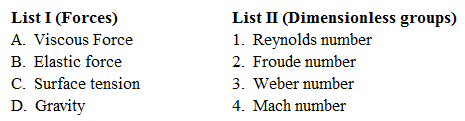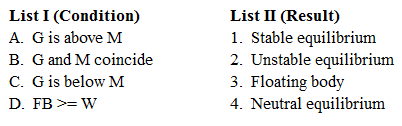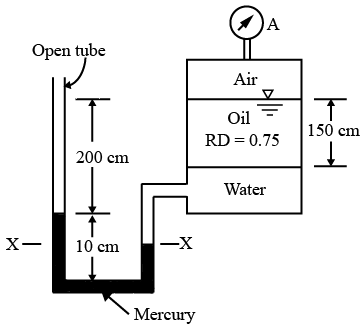# ISRO Scientist or Engineer Mechanical 2007

Instructions

For the following questions answer them individually

Question 21

# A centrifugal pump with peripheral speed ‘V’ was selected fora total lift of 100 m. Actually pump wasto be operated fora total lift of 400 m. The peripheral speed should actually be (other conditions remaining same)Question 22

# The specific speed of pump and turbine respectively are given asQuestion 23

# Match List I with List II and select the correct answerQuestion 24

# Given that$$\alpha_1$$ = nozzle anglen = number of rows of moving blades, in a velocity compounded impulse turbine, the optimum blade speedratio isQuestion 25

# The following terms relate to floating bodies:Centre of gravity ... G, MetaCentre ... M, Weight of floating body ... W, Buoyant force ... Fg, Match List I with List II and select the correct answerQuestion 26

# A pipeline connecting two reservoirs has its diameter reduced by 20% due to deposition of chemicals. For a given head difference in the reservoirs with unaltered friction factor, this would cause a reduction in discharge ofQuestion 27

# An open rectangular box of base 2m $$\times$$ 2m contains a liquid of specific gravity 0.80 up to a height of 2.5 m. If the box is imparted vertically upward acceleration of 4.9m/s$$^2$$, what will be the pressure on the base of the tank?Question 28

# The tank shown in the figure below is closed at top and contains air at a pressure of $$P_A$$. The value of $$P_A$$, for the manometer readings shown will beQuestion 29

# $$P = 2i - 3j, Q = -3i + 4j - 2k$$, and R are in equilibrium, if R isQuestion 30

# A rigid body is rotating with constant angular velocity $$\omega$$ about a fixed axis, if v is the velocity of a point of the body, then curl v =OR